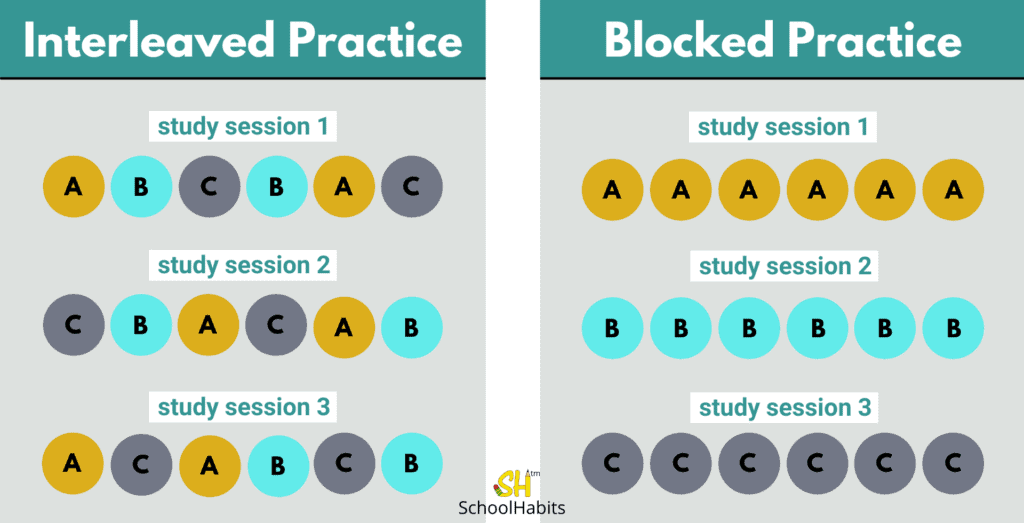# Study techniques: How to use interleaved practice to study

Katie Azevedo

By Katie Azevedo, M.Ed.

The purpose of this post is to teach how to use interleaved practice to study.

Although they may not know it, most students study with a less effective technique called blocked practice. Blocked practice is when a student studies one particular skill, using that skill in isolation on a series of similar problems or questions – all of which require that one skill to solve.

#### In math, blocked practice could look like the following:

A student studies the Pythagorean theorem by using the Pythagorean theorem to solve 10 practice questions, all of which can be solved using the Pythagorean theorem.

#### In English, blocked practice could look like the following:

A student studies subject-verb agreement by finding subject agreement errors in 10 practice questions, all of which contain subject-agreement errors.

### Blocked practice vs. interleaved practice

Blocked practice is helpful and effective for learning how to use a skill or strategy, particularly for athletic training; however, it’s a terrible study technique for learning when to use a skill or strategy, because the student already knows which strategy to use before even reading the problem. The issue lies in the fact that nearly all tests and final exams (including standardized tests like the SAT, ACT, GRE, LSAT, etc.) require students to not only know how to use a strategy, but to determine which strategy to use.

That previous paragraph is very, very important. If you didn’t quite catch that, go back and read that paragraph again.

Instead of using blocked practice to study, students should be using interleaved practice. Interleaved practice is when a student deliberately studies by attempting to answer a variety of question-types, in a random or scrambled order, all of which require various strategies to solve. The critical point here is that the student is learning when to use a particular strategy to solve a problem, not just how to.

In a 2014 study published in the Journal of Educational Psychology, researchers determined that “apart from its superiority to blocked practice, interleaved practice provided near immunity against forgetting.”

Oh.My.Gosh. Immunity against forgetting!? What?! This stuff is no joke.In the above image, A, B and C represent 3 types of practice questions that each require a different strategy to solve.

#### In math, interleaved practice could look like the following:

1. Situation: A student needs to learn the Pythagorean theorem, the distance formula, and the Quadratic Reciprocity Theorem.
2. First, the student should know each of these formulas, perhaps by using blocked practice.
3. Then, the student should scramble the three different types of problem sets, and practice determining which of the three strategies is required to solve each question.

#### In English, interleaved practice could look like the following:

1. Situation: A student needs to learn about subject-verb agreement, non-pronoun agreement, and dangling modifiers. (Sounds like this student is taking the SAT!)
2. First, the student should know how to recognize each type of grammar error in isolation.
3. Then, the student should scramble a variety of sentences, each with one of the three grammar errors (or no error at all) and practice determining which sentences contain which type of errors.

Knowing how to use interleaved practice to study is kind of a super power. This study technique works especially well for unit tests, final exams, and standardized tests – all of which require students to look at a question, figure out what it’s asking, determine the correct strategy to use – and only then, solve it.

Better yet, use interleaved practice with these other study tips for best results.